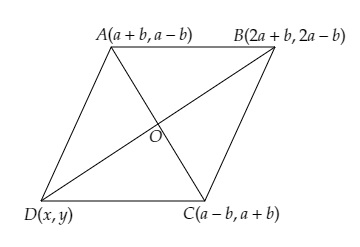# Three vertices of a parallelogram are $(a + b, a ‚Äì b), (2 a + b, 2a ‚Äì b), (a ‚Äì b, a + b)$. Find the fourth vertex.

Given:

Three vertices of a parallelogram are $(a + b, a – b), (2 a + b, 2a – b), (a – b, a + b)$.

To do:

We have to find the fourth vertex.

Solution:

Let the coordinates of the three vertices are $A(a + b, a – b), B(2 a + b, 2a – b), C(a – b, a + b)$.
Let the fourth vertex be $D(x,y)$ and the diagonals $AC$ and $BD$ bisect each other at $O$.This implies,

$\mathrm{O}$ is the mid-point of $\mathrm{AC}$.

The coordinates of $\mathrm{O}$ are $(\frac{(a+b)+(a-b)}{2}, \frac{(a-b)+(a+b)}{2})$

$=(\frac{2a}{2}, \frac{2a}{2})$

$=(a,a)$

$\mathrm{O}$ is the mid-point of $\mathrm{BD}$.

The coordinates of $\mathrm{O}$ are $(\frac{2a+b+x}{2}, \frac{2a-b+y}{2})$

Therefore,

$(a,a)=(\frac{2a+b+x}{2}, \frac{2a-b+y}{2})$

On comparing, we get,

$\frac{2a+b+x}{2}=a$

$2a+b+x=2(a)$

$x=2a-2a-b=-b$

Similarly,

$\frac{2a-b+y}{2}=a$

$2a-b+y=2(a)$

$y=2a-2a+b$

$y=b$

Therefore, the coordinates of the fourth vertex are $(-b,b)$.

Updated on: 10-Oct-2022

31 Views